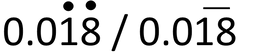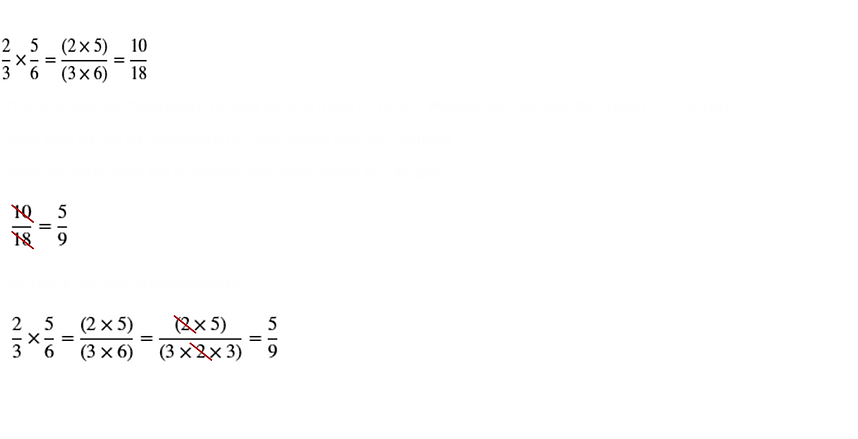top of page#### 1.1 Integers, Fractions and Decimals

In this section we will briefly review how to carry out calculations involving integers, fractions and decimals. If you believe you are well versed in this section then please proceed on to the more advanced sections. However a quick review will not hurt you.

## Mathematical Words and Expressions

Let's go over some terms that will help us understand how numbers are categorized in mathematics. I do not think it is important for you to memorize these terms but it might better help you understand mathematical concepts.

Whole Number - This is any number from 0 to infinity that does not need to be expressed in the form of a fraction or decimal. It is also important to note that negative numbers are not  whole numbers. 0,1,2,10,50,100000,11125151515 are whole numbers. 1.2, 0.365, 1/8, -20, -10, -100000 are not whole numbers.

Natural Number - This is any whole number except for 0. So you could also say that all natural numbers are whole numbers but not all whole numbers are natural numbers since 0 is not a natural number.

Even Number - This is any natural number that can be divided exactly by 2. So 10, 90, 100, 666 are even numbers.

Odd Number - This is any natural number that cannot be divided exactly by 2. So 3, 7, 49, 789 are odd numbers.

Integer - These numbers include all the whole numbers and the negative of those whole numbers. The whole numbers are known as positive integers. The negative of the whole numbers are known as negative integers.

Now, is 0 a positive integer or negative integer? It is neither positive nor negative but since it is a whole number, it is considered to be an integer.

Therefore, 0, 2, -2, 50, -11125151515 are integers. But -1.2, -0.365, 0.365, 1/8 are not integers.

Multiple - The multiple of a number is the answer you get when you multiply that number by an integer.

So what is the multiple of 5? If we take an integer say 0 and multiply by 5 we get 0. So 0 is a multiple of 5.

Is that the only multiple of 5? No, since there is an infinite amount of integers there are an infinite amount of multiples for 5. Therefore 10,15,-10,-35, etc are all multiples of 5.

What is a multiple of 3.5? If we multiply it by 3 the answer we get is 10.5. So 10.5 is a multiple of 3.5. As you can see, if the number is a fraction or decimal, the multiple of that number does not have to be an integer.

Factor - A factor of a number is what you can multiply with another number to get that number exactly. What are the factors of 16? Well to get 16 you can multiply 4 x 4. So 4 is a factor of 16. Or you can multiple 8 x 2. Therefore, 8 and 2 are also factors of 16. You should notice that 16 is a multiple of 2, 4 and 8. Unlike multiples, factors are always integers and they cannot be decimals or fractions. You should have also noticed that even though a number can have an infinite number of multiples, a number can only have a set number of factors.

3 x 15 = 45

3 is a factor or 45

15 is a factor of 45

45 is a multiple of 3

45 is a multiple of 15

Prime Number - This is any natural number that is only divisible by itself and 1. In other words, it only has two factors: itself and 1. Example are 2, 7, 3, 107, 433, 5, etc.

## Decimals

Let's just quickly review how to do some simple calculations with decimals.

##### Multiplying and Dividing Decimals

Working with decimals is not all that hard. Sometimes the decimal point might confuse you especially when you don't where exactly to put it in your final answer.

When I am multiplying, first of all I just ignore the decimal point and do the calculation. So let's look the following problem:

Example 1:

0.25 x 0.3

If you focus on the decimals it gets confusing. So lets temporarily get rid of the decimals. For 0.25 we can move the decimal point twice to the right to get a whole number. For 0.3 we can move it once to the right to get a whole number. We have moved the decimal points 3 times in total to get:

25 x 3

The answer for this is 75. Now we can deal with the decimals. We moved the decimals 3 times in total, which means the decimal point for our answer must be moved back 3 times. Since we are multiplying, the decimal point must be moved back to the left. Starting from 5 and moving the point 3 times we get 0.075. Therefore the answer is:

0.25 x 0.3 = 0.075

Easy. Lets look at one more example:

Example 2:

0.0025 x 0.003

move point 4 times to get 25 and 3 time to get 3 for total of 7 times

25 x 3 = 75

move decimal back to the left for a total of 7 times

0   .   0   0   0   0   0   7   5

Division is also similar but you have to be more careful when moving the decimal points. Lets look the following problem:

Example 3:

0.27 / 0.3

For division, the decimal point can only be moved the same number of times for both numbers. So if you move it once to the right for 0.27 to get 2.7 then the it can only be moved once to the right for 0.3 as well to get 3. If you move it twice to the right for 0.27 then it must be moved twice to the right for 0.3 as well.

When dividing decimals, it is much easier to do so if the denominator is a whole number without a decimal.

Therefore to solve this, let's move it once to the right so that we get a  whole number in the denominator:

2.7 / 3 = 0.9

The answer is 0.9. Since the numerator has 1 d.p, then the answer will too.

Let's look at one more:

0.0154 / 0.7
move the decimal point 1 time to the right so that we could make 0.7 a whole number

0.154 / 7

0 . 0 2 2

0 . 1 5 4

7

1 4

1 4

1 4

0

##### Convert Decimals to Fractions

Converting from decimal to fraction and vice versa is straight forward. However, you have to make sure that the decimal being converted is a terminal decimal not a recurring decimal. A recurring decimal is a number where the digits just keep on repeating itself forever; whereas, a terminal decimal is one with a finite set of numbers.

If a decimal is recurring it is shown by placing dot on the first and last digit that keeps repeating or by placing a line of the section that keeps repeating. For example:These numbers cannot be expressed as perfect fractions. For terminal decimals you just need to know the position of the digits that come after the decimal point. The first number after the decimal point is in the tenths position. Next to it is the hundredths and so on as shown below:Let's look at the examples below. For 0.23, the farthest right number is 3 and it is in the hundredths positions. This means 23 has to be divided by 100 to get the fraction. Similarly for 0.250, 0 is the farthest right and in the thousandths position. This means that 250 has to be divided by 1000. It also very important to simplify your answers. So in this both 25 and 100 can be divided by 5 to reach the simplest fraction 1/4.Even if you have digits on the left hand side of the decimal point, converting to fraction is still the same process as above. Look at the farthest number to the right of the point and determine its position. For 2.15, the position of 5 is hundredths. Therefore, you take the whole number (215) without the decimal point and divide by 100 to get the fraction. Then always make sure the fraction is in its simplest form.Or you could first convert these type of decimal numbers to a mixed fraction. You take the number or numbers on the left of the point as a whole number and write it down. Then you consider the numbers on the right of the point and convert it to a fraction like we did above and write that number next the whole number to get a mixed fraction. A mixed fraction just like the name implies is a number that has a mix of a whole number and a fraction togther.When simplifying mixed fraction you only have to simply the fraction without doing anything to the whole number.

A mixed number can be converted to just a fraction. This type of a fraction is called an improper fraction because the numerator is larger than the denominator. To convert a mixed number to an improper fraction you first multiply the denominator and the whole number together, then add that value to the numerator. This value is the numerator and the denominator is the same as that of the mixed number.## Fractions

##### Multiplying and Dividing Fractions

Let's quickly review fractions.

Multiplying fractions is very easy since it's straight forward. Just multiply the numerators togther and then the denominators together.Division is not so straight forward:When you have mixed fractions, they have to be converted to improper fractions before calculations can be performed. This is explained above. To convert you just multiply the denominator with the whole number and then add that answer to the numerator; the answer to this will be your new numerator and the denominator is still the same:Before adding or subtracting fraction you must make sure the denominator are equal. If they are not equal, they should by manipulated and made equal.Lets look at the above example. The denominator are not equal. To make them equal we have to find a multiple of both 5 and 8 has. If you look in the times table or use your head it is clear that 40 is the LCM.

For 5 to turn into 40 you have to multiply 5 by 8. For 8 to turn into 40, you have to multiply it by 5. Always make sure that if you are changing the denominator by multiplying then you must do the same for the numerator. Therefore, you multiply both the numerator and denominator by the same factor. Finally you can add or subtract only the numerator.

More examples are shown below:As you can see here, once again mixed fractions must be converted to improper fractions before calculating the answer.

bottom of page